#### 作品展示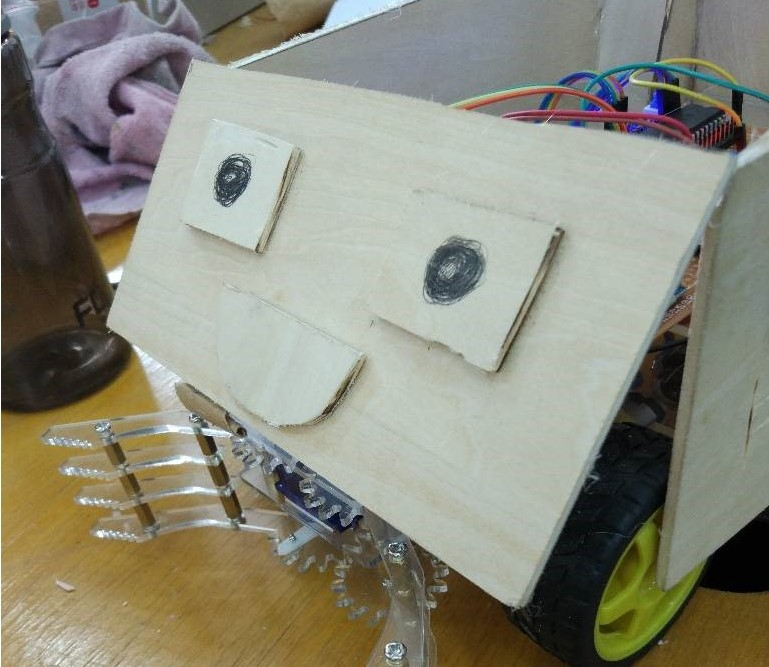#### 代码实现

``````#include <reg52.h>
#define uchar unsigned char
#define uint unsigned int
void delay(uint i);
sbit z1 = P1 ^ 0;
sbit z2 = P1 ^ 1;
sbit y1 = P1 ^ 2;
sbit y2 = P1 ^ 3;

uint aa;
sbit PWMOUT = P0 ^ 1;

unsigned char angle = 0;
unsigned char HighRH = 0;
unsigned char HighRL = 0;
unsigned char LowRH = 0;
unsigned char LowRL = 0;

void ConfigPWM(unsigned int fr, unsigned char dc);
void ClosePWM();

void main()
{
unsigned int i;
TMOD = 0X20;
IE = 0x82;
TH1 = 0xfd;
TL1 = 0xfd;
TR1 = 1;
SM0 = 0;
SM1 = 1;
REN = 1;
ES = 1;
ConfigPWM(50, 9);

while (1)
{
if (aa == 0x30)
{
ConfigPWM(50, 13);
for (i = 0; i < 10000; i++);
}
else if (aa == 0x31)
{
ConfigPWM(50, 7);
for (i = 0; i < 8000; i++);
}
else if (aa == 0x12)
{
z1 = 1;
z2 = 0;
y1 = 1;
y2 = 0;
}
else if (aa == 0x13)
{
z1 = 0;
z2 = 1;
y1 = 1;
y2 = 0;
}
else  if (aa == 0x14)
{
z1 = 1;
z2 = 0;
y1 = 0;
y2 = 1;
}
else  if (aa == 0x15)
{
z1 = 0;
z2 = 1;
y1 = 0;
y2 = 1;
}

else
{
z1 = 0;
z2 = 0;
y1 = 0;
y2 = 0;
}

delay(10);
}

}
void ConfigPWM(unsigned int fr, unsigned char dc)
{
unsigned long tmp;
unsigned int high, low;
tmp = 11059200 / 12 / fr;
high = (tmp * dc) / 100;
low = tmp - high;
high = 65536 - high + 12;
low = 65536 - low + 12;
HighRH = (unsigned char)(high >> 8);
HighRL = (unsigned char)high;
LowRH = (unsigned char)(low >> 8);
LowRL = (unsigned char)low;
TMOD &= 0xF0;
TMOD |= 0x01;
TH0 = HighRH;
TL0 = HighRL;
ET0 = 1;
TR0 = 1;
PWMOUT = 1;

}
void InterruptTimer0() interrupt 1
{
if (PWMOUT == 1)
{
TH0 = LowRH;
TL0 = LowRL;
PWMOUT = 0;
}
else
{
TH0 = HighRH;
TL0 = HighRL;
PWMOUT = 1;
}
}
void Uart(void) interrupt 4
{
EA = 0;
if (RI == 1)
{

aa = SBUF;
RI = 0;

}
EA = 1;
}
void delay(uint i)
{
uchar x, j;
for (j = 0; j < i; j++)
for (x = 0; x <= 148; x++);
}
``````

#### 作品陈列

我们的射手小车，采用诸葛连弩的结构模型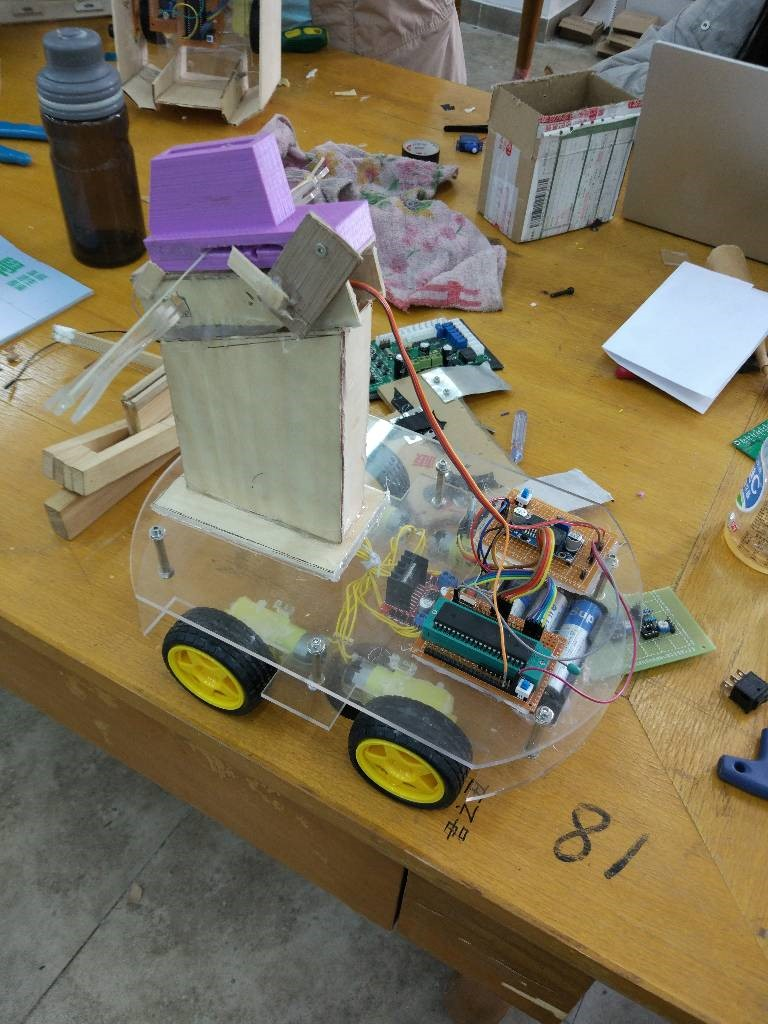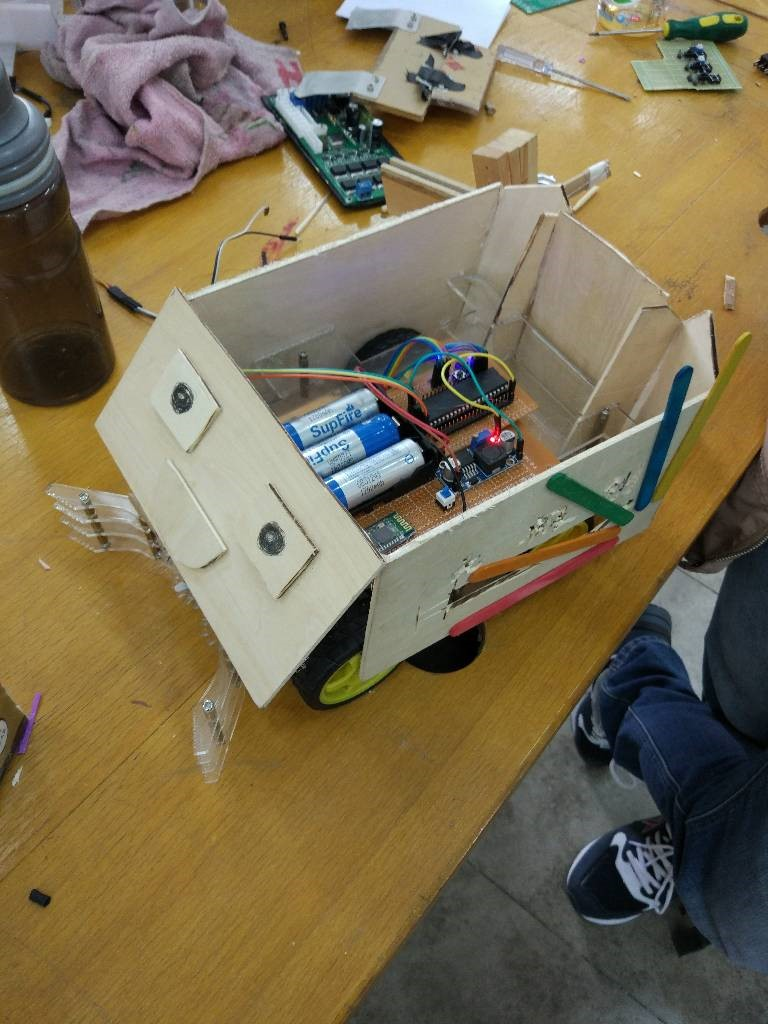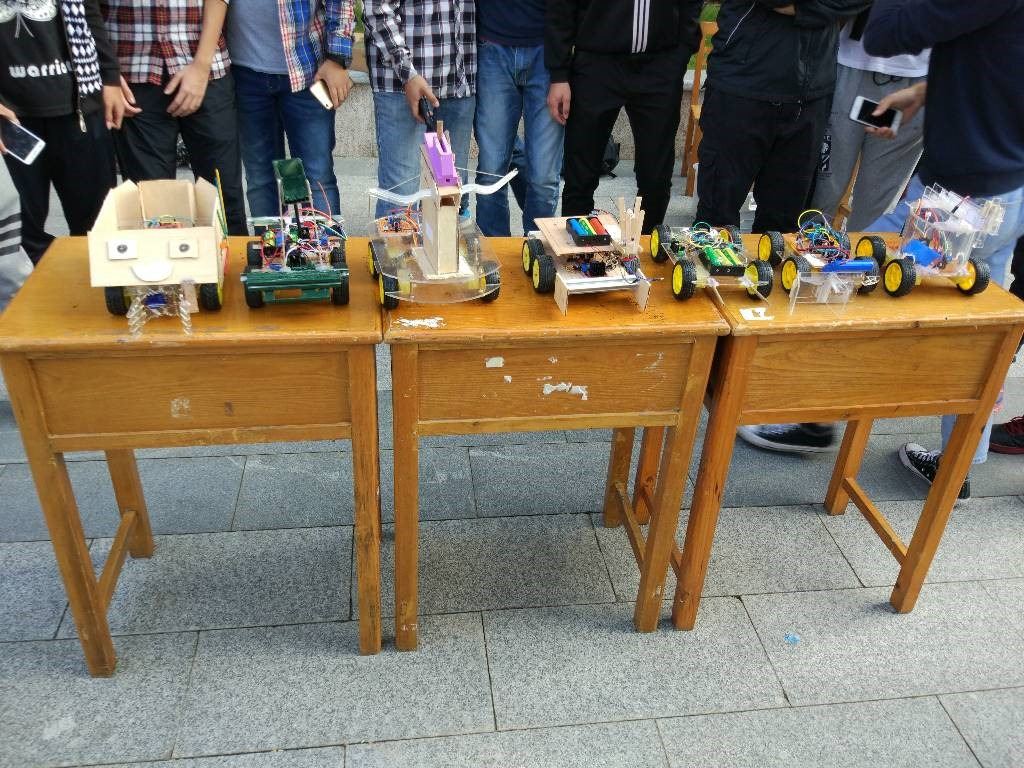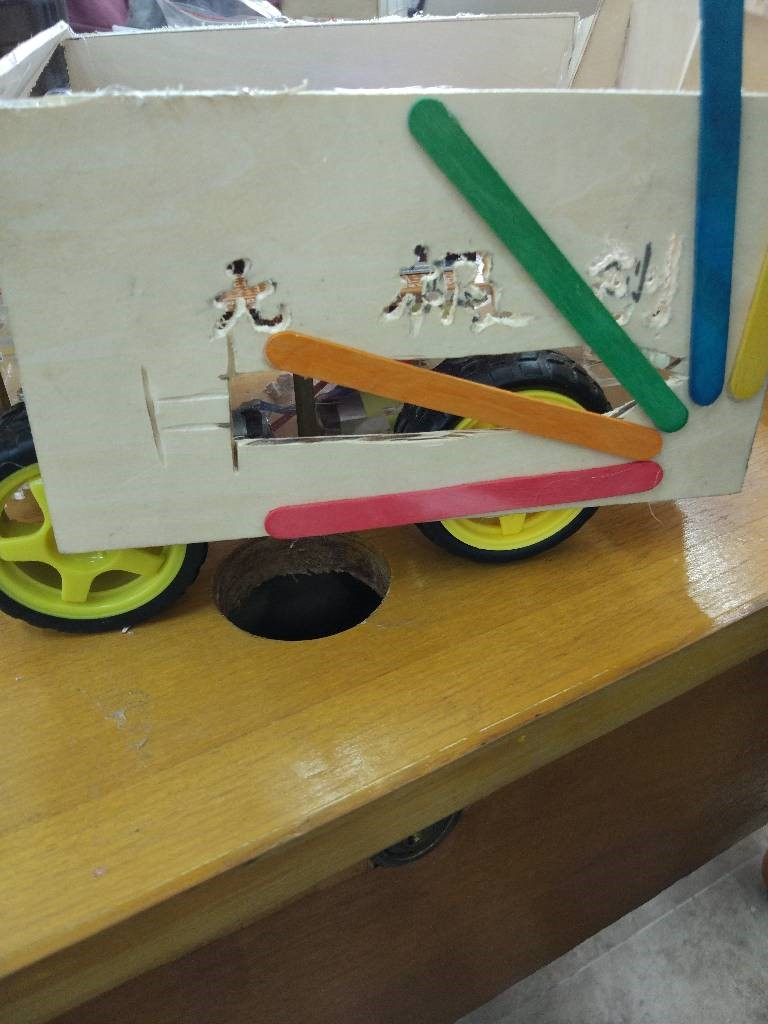``````                               感谢陪我走过大一风风雨雨兄弟们
一起笑，一起闹，一起熬夜
为比赛而努力的我们
加油
远方路很长
且行且珍惜
————无极创
``````